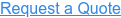My parents were fond of saying, “There is only so much meat on the bone.” No matter how hungry we children were, there was a limit as to what meat we could get from gnawing a bone. The same is true for coils. No matter how hard we wish for more, a coil can only transfer a limited amount of heat. Why is that?

The answer is a combination of several heat transfer parameters and engineering principles, but it boils down to two main factors: heat capacity rate and approach. Understanding these two yields a better grasp of what works and what doesn’t. A simple example can help explain why.

Suppose a coil needs to cool 8,000 scfm of dry air at 120°F using 26 gpm of cooling water coming from a lake that yields a design entering water temperature of 90°F. The first, readily apparent observation is that it is not possible to cool the air below 90°F, nor is it possible to heat the water above 120°F. Therefore, the maximum temperature change (ΔTmax) for either the air or the water is limited to 30°F.

Specific heat

A coil is used to get the heat from the air to the water without mixing them together. In a well-insulated system, the amount of heat transferred from the air (Q air) and through the coil must equal the amount of heat transferred into the water (Q H2O). Both the air and water have a thermal characteristic used to quantify how much heat energy each can absorb or give up in order to change its temperature by one degree.

This property is specific heat (cp):

For dry air, the specific heat (cp-air) is 0.24 Btu/lbm-°F.

For water, the specific heat (cp-H2O) is 1.0 Btu/lbm-°F.

Heat capacity rate

Heat transfer is equal to (mass flow rate x specific heat x change in temperature). Since Q air = Q H2O and the maximum possible temperature change for both the air and the water is the same, the maximum amount of heat that can be transferred is limited by the medium with the lower value of (mass flow rate x specific heat). This is the heat capacity rate (C). In the example:

Heat capacity rate of air (C air)        = (8,000 scfm)(.075 lbm/ft3)(60 min/hr)( 0.24 Btu/lbm-°F)

= 8,640 Btu/hr/°F

Heat capacity rate of water (C H2O) = (26 gpm / 7.48 gal/ft3)(62 lbm/ft3)(60 min/hr)(1.0 Btu/lbm-°F)

= 12,930 Btu/hr/°F

As is often the case, the air has the lower heat capacity rate, limiting the amount of heat that can be transferred. In this example, no matter how much the water flow rate increases, the air side restricts the cooling capacity. Want more cooling? You need more air.

So how much cooling can the coil deliver?

In the example, the theoretical maximum possible heat transfer is determined from the air side (the medium with the lower heat capacity rate):

(Q air)    = C air x ΔTmax

= (8,640 Btu/hr/°F)(30°F)

= 259,200 Btu/hr

Approach

Unfortunately, a coil with an infinite amount of heat transfer surface (i.e., the fin-tube “bones” of the coil) is required to achieve this amount of thermal heat transfer (i.e., the “meat”). More heat transfer surface is required the closer the leaving air temperature gets to the cooling water temperature. The closeness of the leaving air temperature to the entering water temperature (or the leaving water temperature to the entering air temperature, when the water has the lower heat capacity rate) is the “approach”. The closer the approach, the larger a coil is needed.

When initially sizing a cooling coil, a 6°F approach is a nice place to start in order to obtain a reasonable selection. However, there is no definitive value. All coil sizing parameters can be adjusted to produce a selection that best balances thermal performance requirements with cost constraints. Fortunately, Super Radiator Coils’ Enterprise software program accommodates simple iterations to quickly zero in on a coil’s optimal size.

While there are other parameters to consider when sizing coils, a basic understanding of heat capacity rate and approach gives insight into why more “bones” may be needed to get more “meat”. Otherwise, you may find that “you can’t get there from here” – another favorite idiom of my parents. Fortunately, when you need help getting there, a Super Radiator engineer is always ready to support your project needs and assist with challenging specification requirements. If it has to be perfect, it has to be Super!

Don’t get left out in the cold when it comes to heat transfer information. To stay up to date on a variety of topics on the subject, subscribe to The Super Blog, our technical blog, Doctor's Orders, and follow us on LinkedIn, Twitter, and YouTube.

## We’re fluent in answers

Need someone who understands your language? Simply answer a few questions and we’ll get our engineers on it.